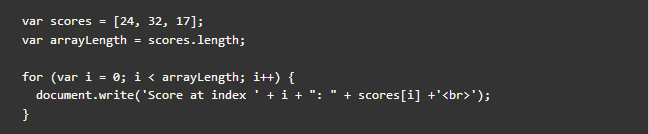#### For Loop

Another kind of loops is For Loop. You use a for loop if you need to run code a specific number of times. For loops have mainly 4 parts:

1. Initialization - A value is set initially which is the starting value of the counter
2. Condition - Tests whether the given conditional statement is true or not
3. Iteration - Statement which increments or decrements the counter
4. Body - Code inside the for loop. Body is only executed if condition statement (#2) is true

Let's look at an example:A for loop is often used to loop through the items in an array. In this example, the scores are stored in an array called scores. The total number of items in the array is stored in a variable called array length. This number is obtained using the length property of the array. counter is stored in a variable called i. The loop starts with the for keyword, then contains the condition inside the parentheses. As long as the counter is less than the total number of items in the array, the contents of the curly braces will continue to run. Each time the loop runs, items in the scores array is printed to the page.

Remember: Array index starts at 0 and not 1.

NEXT LESSON